# Overview: Data Calculations

With Data Calculations you can combine data from any Data Source in an equation to create new Calculated Metrics. Some popular use cases for Data Calculations include calculating Conversion Rates, ROI, ROAS, and more.

# How to Access Data Calculations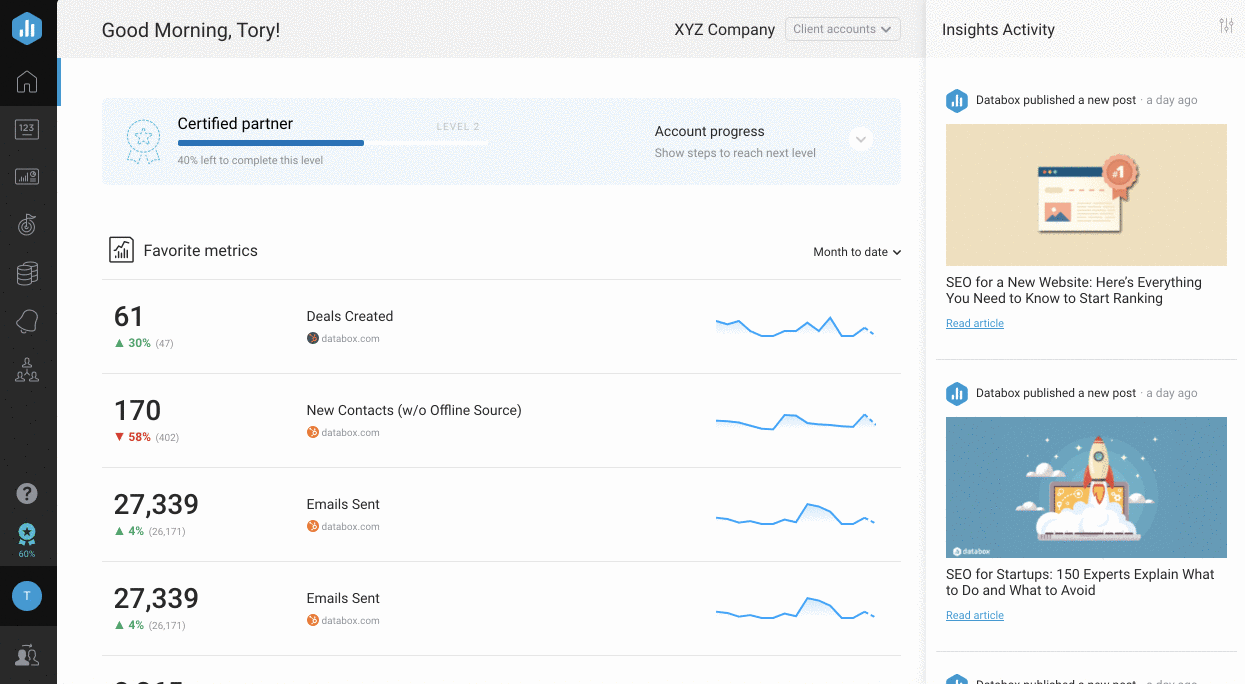Navigate to  Metrics > Data Calculations to access Data Calculations.

Data Calculations is available on the Professional and Performer plans. Request a trial of Data Calculations by following these steps.

# How to Create a Calculated Metric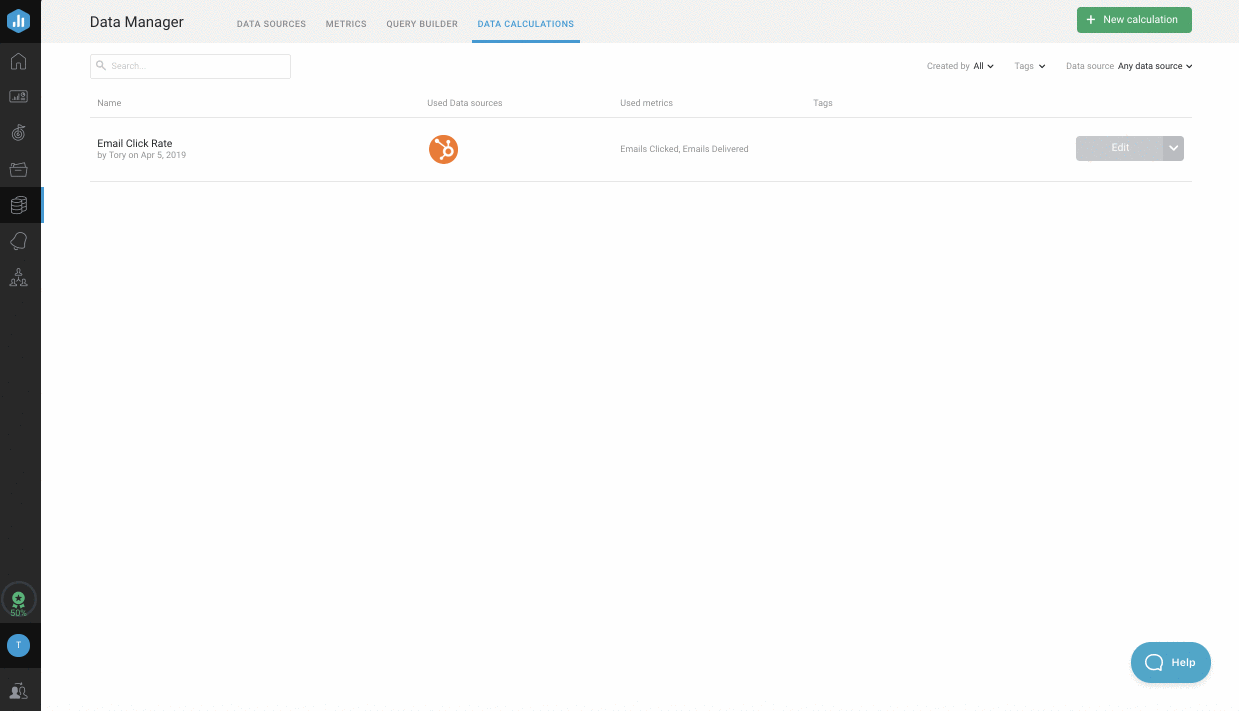1
Enter a name for your Calculated Metric in the Calculated Metric Name text box.
2
Click on variable A to open the first Metric selection window. Select the first Data Source and Metric that you want to use in your equation. To manually enter a value into the equation, select Set a fixed value.
On the right side of the Data Calculations window, you can see a Data Preview for your Calculated Metric. The Date Ranges available for the Calculated Metric are dependent on the Date Ranges available for the individual Metrics used in the calculation.
3
Click on the blue + operator to update the equation to add, subtract, multiply or divide the next variable. Parenthesis can be used to adjust the order of operations.
4
Click on variable B to open the next Metric selection window. Select the next Data Source and Metric that you want to use in your equation. To manually enter a value into the equation, select Set a fixed value.
5
Repeat steps 4 and 5 with the remaining variables until your equation is complete.
6
Update the Format and Scale for the Calculated Metric at the bottom of the Data Preview. Learn more about Format and Scale here
7
Click Save to save the Calculated Metric in your Account.

# How to calculate Metrics with Units

Some Metrics are stored with associated units. Typically, these are currency Metrics (like "Amount Spent" from Facebook Ads or "Spend" from Google Ads) where the unit is referencing the currency type (i.e., USD, EUR, AUD, etc.).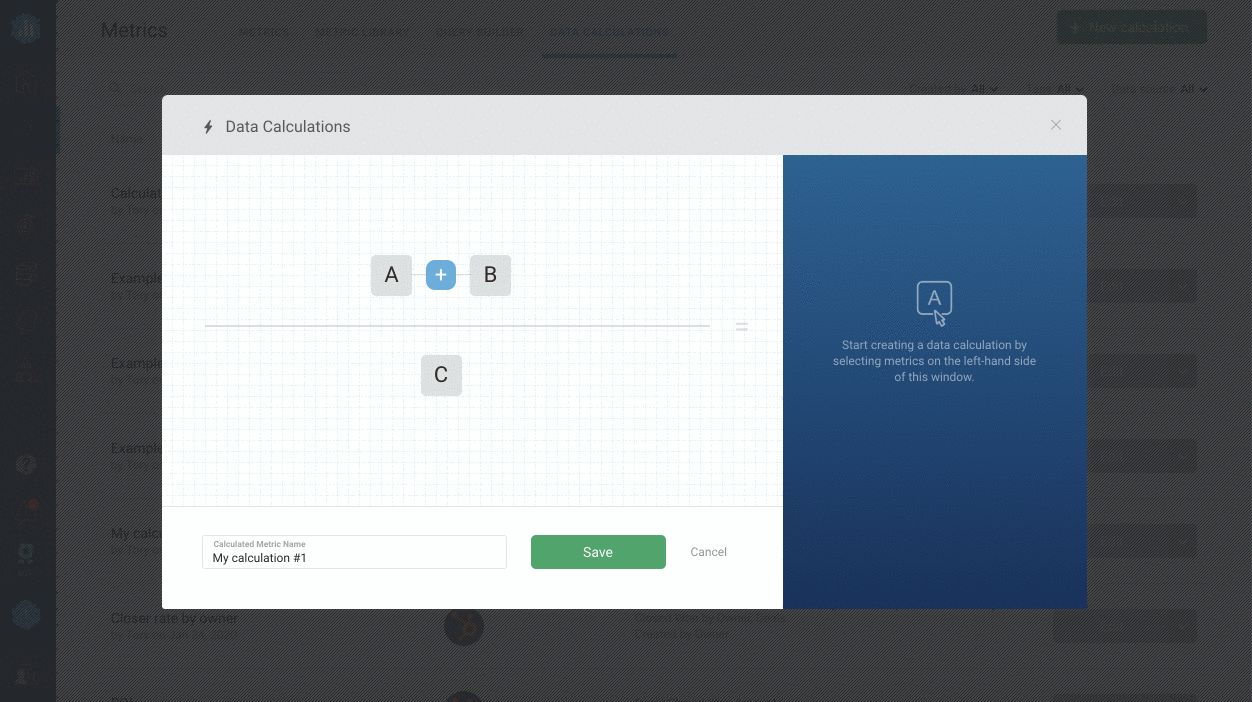When creating Calculated Metrics using Metrics with Units, it is important that you select the Unit for each Metric in the calculation.  If not, the intended calculation may not be executed correctly.
Before saving the Calculated Metrics check at Data Preview on the right side of the Data Calculations window if the Unit is being displayed. If the Unit is not being displayed, update the Format for the Calculated Metrics at the bottom of the Data Preview. You can choose between Prefixed \$, Prefixed £, Prefixed €, Prefixed A\$, Prefixed currency, Postfixed currency, Prefixed unit, Postfixed unit, or add a New Custom Format. Learn more about how to add a custom Format  here.
You must also select the Unit in the Designer when adding the Metric to a Databoard. If not, multiple values may be displayed on your Databoard.

# How to calculate Attributed Metrics

If an Attributed Metric with a Dimension selected is calculated with another Attributed Metric with a Dimension selected, the calculation will be performed on the Dimensions. The Calculated Metric created from this will be a Standard Metric (no Dimensions).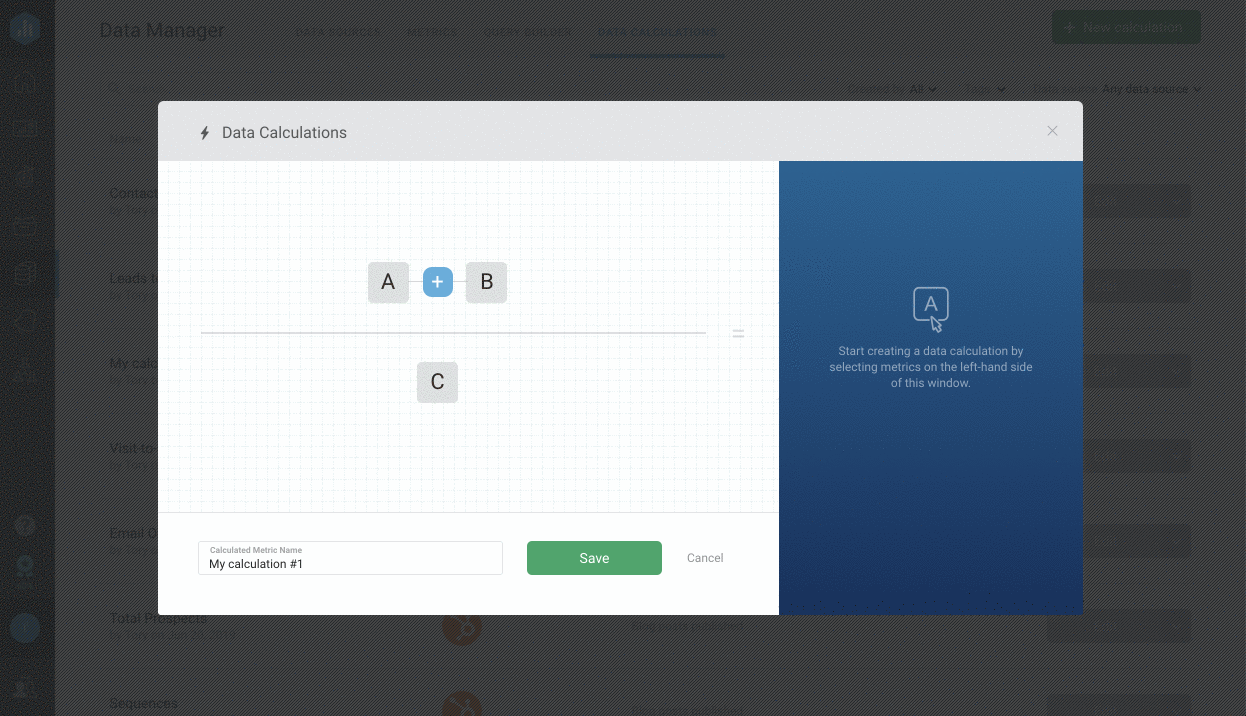For example, the Organic Session-to-Lead Conversion Rate can be calculated by doing "Leads by Source" with the "Organic" Dimension selected / "Sessions by Source" with the Organic Dimension selected. The resulting Calculated Metric now stores data for "Organic Session-to-Lead Conversion Rate."

If an Attributed Metric with no Dimension selected is calculated with a single value (whether that be a Standard Metric, an Attributed Metric with 1 Dimension selected, or a fixed number), the calculation will be executed individually for every Dimension. The Calculated Metric created from this would be an Attributed Metric.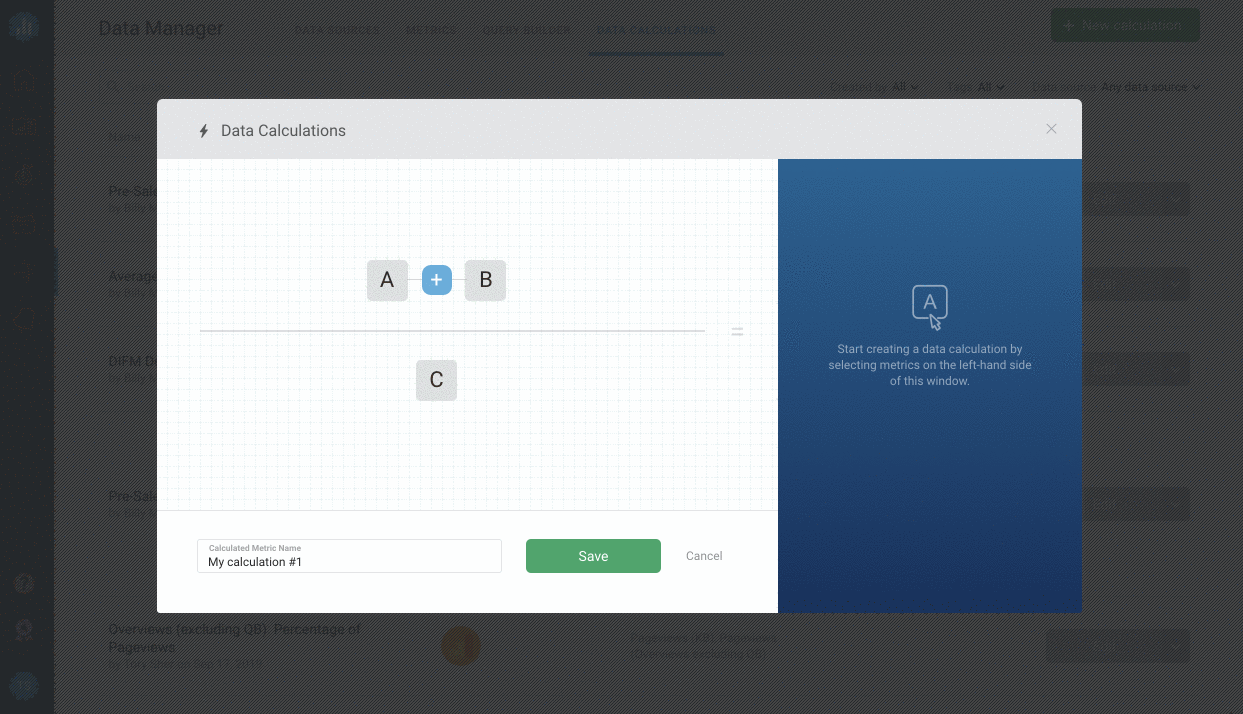For example, you may spend \$100 on every Facebook Ads Campaign that isn't tracked in Facebook Ads itself. To account for this, you can calculate the following: "Spend by Campaign" (no Dimension selected) + 100. This will add 100 to every Campaign.

If an Attributed Metric with no Dimension selected is calculated with another Attributed Metric with no Dimension selected, the calculation will be performed only between identical Dimensions. If there are Dimensions that are not found in both Attributed Metrics, no calculation will be performed on them.

The Calculated Metric created from this would be an Attributed Metric as well.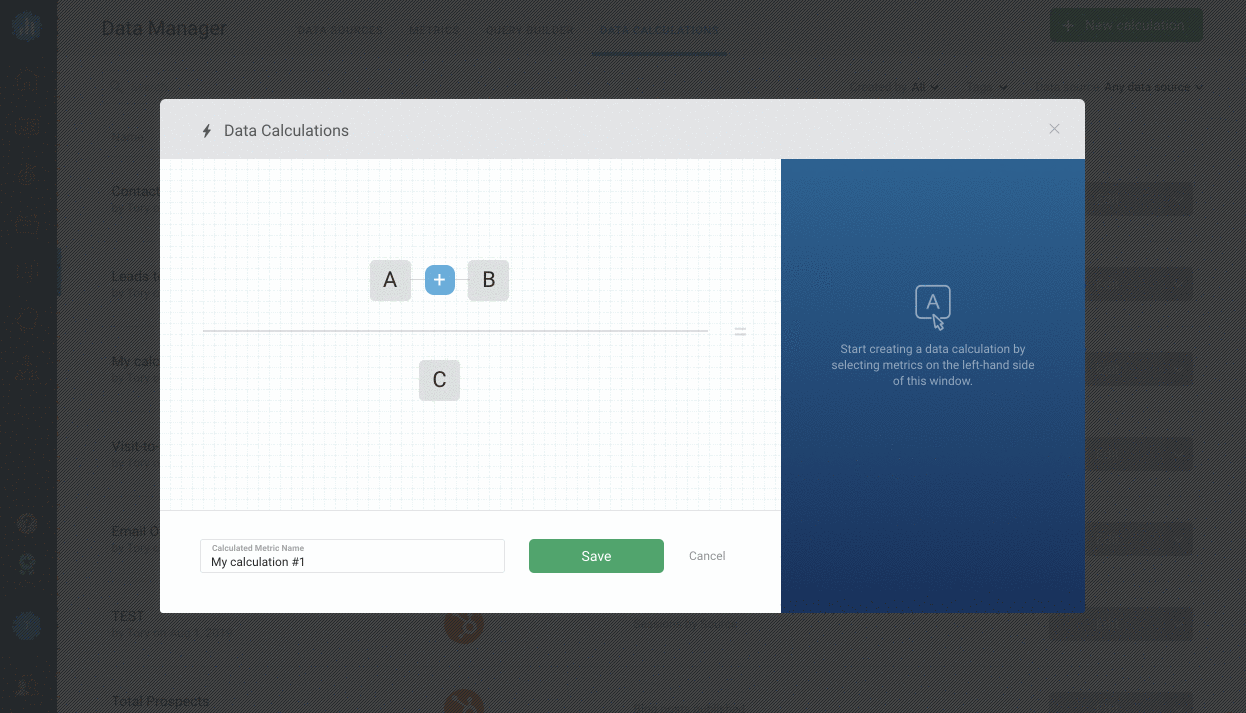For example, you can use this strategy to calculate Email Open Rate for every individual Email. To do this, you would calculate “Emails Opened by Email Title (no Dimension selected)” / “Emails Sent by Email Title (no Dimension selected)“. The calculation will be performed on identical Dimensions, so the resulting Calculated Metric now stores data for “Email Open Rate by Email Title.”

# How to view Calculated Metrics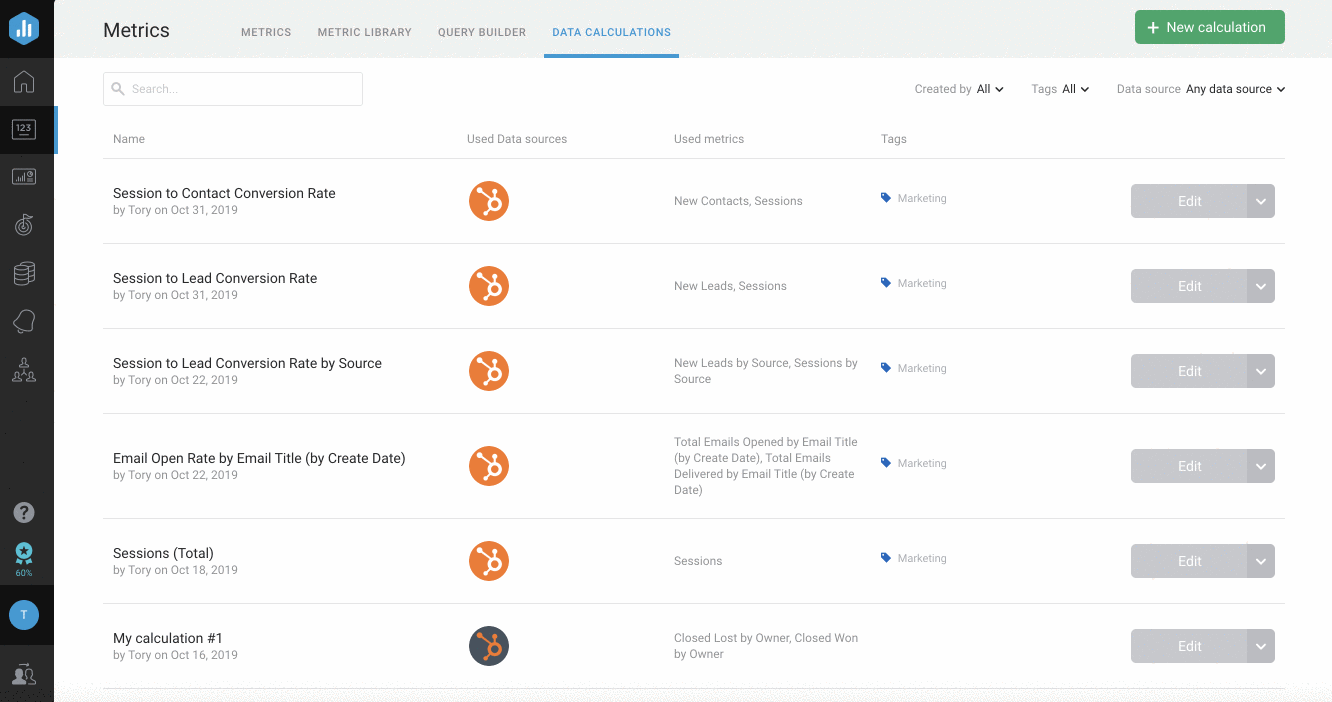Use the Created ByTags, and Data Source drop-downs in the top right of the Data Calculations page to view significant Calculated Metrics.

From the  Data Source drop-down, you can view the number of Calculated Metrics that have been built for each Data Source.

# How to Add a Calculated Metric to a Databoard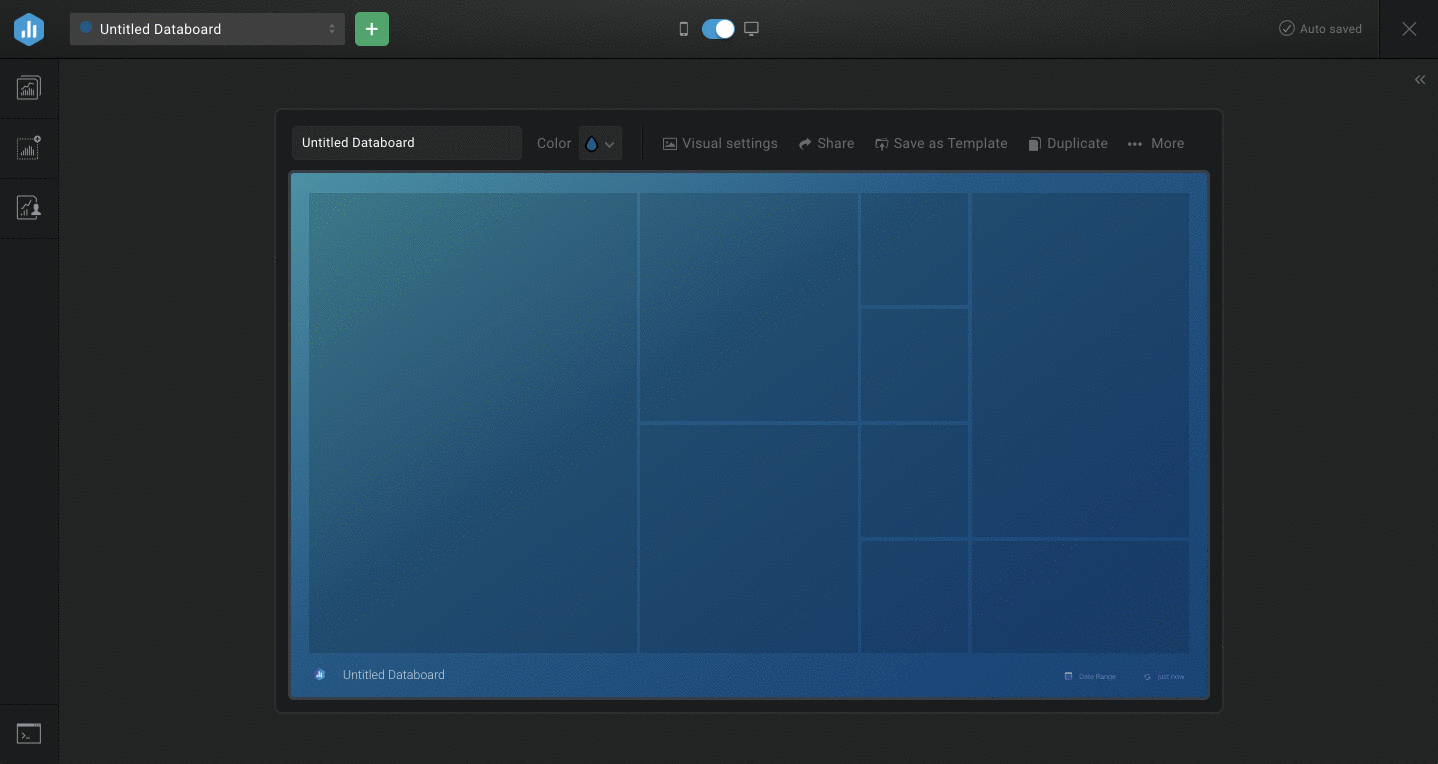1
Open the selected Databoard in the Databox Designer, or create a new Databoard.
2
Drag and drop a blank Datablock onto the Databoard from the Visualization Library.
3
Select the appropriate Data Source in the righthand Property Manager. This must be the same Data Source that was selected when creating the Calculated Metric. If data from multiple Data Sources are used in the Data Calculation, select any of the Data Sources that were used in the equation.
4
Select the Calculated tab at the top of the Metric drop-down list.
5
Select the Calculated Metric name to populate the Datablock.